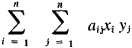Bilinear Form

(redirected from Antisymmetric bilinear form)

bilinear form

[¦bī‚lin·ē·ər ′fȯrm]
(mathematics)
A polynomial of the second degree which is homogeneous of the first degree in each of two sets of variables; thus, it is a sum of terms of the form aijxiyj, where x1, … , xm and y1, … , yn are two sets of variables and the aij are constants.
More generally, a mapping ƒ(x, y) from E × F into R, where R is a commutative ring and E × F is the Cartesian product of two modules E and F over R, such that for each x in E the function which takes y into ƒ(x, y) is linear, and for each y in F the function which takes x into ƒ(x, y) is linear.

Bilinear Form

a form—that is, a homogeneous polynomial—of the second degree from two groups of variables x1, x2, . . . , xn and y1, y2, . . . , yn nof the formFor example, axy is a bilinear form of the variables x and y, and a11x1y1 + a21x2y1 + a22x2y2 is a bilinear form of the variables x1, x2, and y1, y2. A bilinear form is a particular type of quadratic form.

Site: Follow: Share:
Open / Close